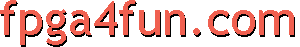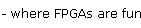## Verilog HDL tips and tricks

This page is not really a tutorial, but a list of sample code that you can study and refer to when you start writing Verilog HDL.
##### Bitwise operators

Verilog operators "&" ("and") and "|" ("or") can be applied to a bus. That allows to "gate" all the individual signals of a bus together.

 wire [7:0] my_bus; // these 2 statements are equivalent wire my_bus_is_all_1s = (my_bus==8'hFF); wire my_bus_is_all_1s = &my_bus; // these 2 statements are equivalent wire my_bus_is_all_0s = (my_bus==8'h00); wire my_bus_is_all_0s = ~|my_bus; // these 2 statements are equivalent wire my_bus_is_non_0 = (my_bus!=8'h00); wire my_bus_is_non_0 = |my_bus;

##### Continuous vs. procedural assignment

Here're 3 different ways to write a 2-to-1 mux.

 wire a, b, c; // This continuous assignment wire my_mux = (a ? b : c); // is equivalent to this procedural assignment reg my_mux; always @(a or b or c) begin   case(a)     1'b1: my_mux = b;     1'b0: my_mux = c;   endcase end // and this one too reg my_mux; always @(a or b or c) begin   if(a)     my_mux = b;   else     my_mux = c; end

##### Bits concatenation
 wire [7:0] my_bus = {2'b01, 4'hF, 1'b1, 1'b0}; wire this_signal_is_true = (my_bus==8'b01111110);

##### Bits replication
 wire [7:0] my_bus = {4{2'b01}}; wire this_signal_is_true = (my_bus==8'b01010101);

 wire [31:0] myvalue = 32'h76543210; wire [3:0] thisis4 = myvalue[19:16]; wire [3:0] thisis4too = myvalue[19-:4]; wire [3:0] thisis4also = myvalue[16+:4];

##### Testbench
```reg clk;

initial    // clock generation
begin
clk = 0;
forever #10 clk = ~clk;
end

initial
begin
@(posedge clk);
while(value==0) @(posedge clk);
repeat(100) @(posedge clk);

\$stop;
\$finish;
end
```